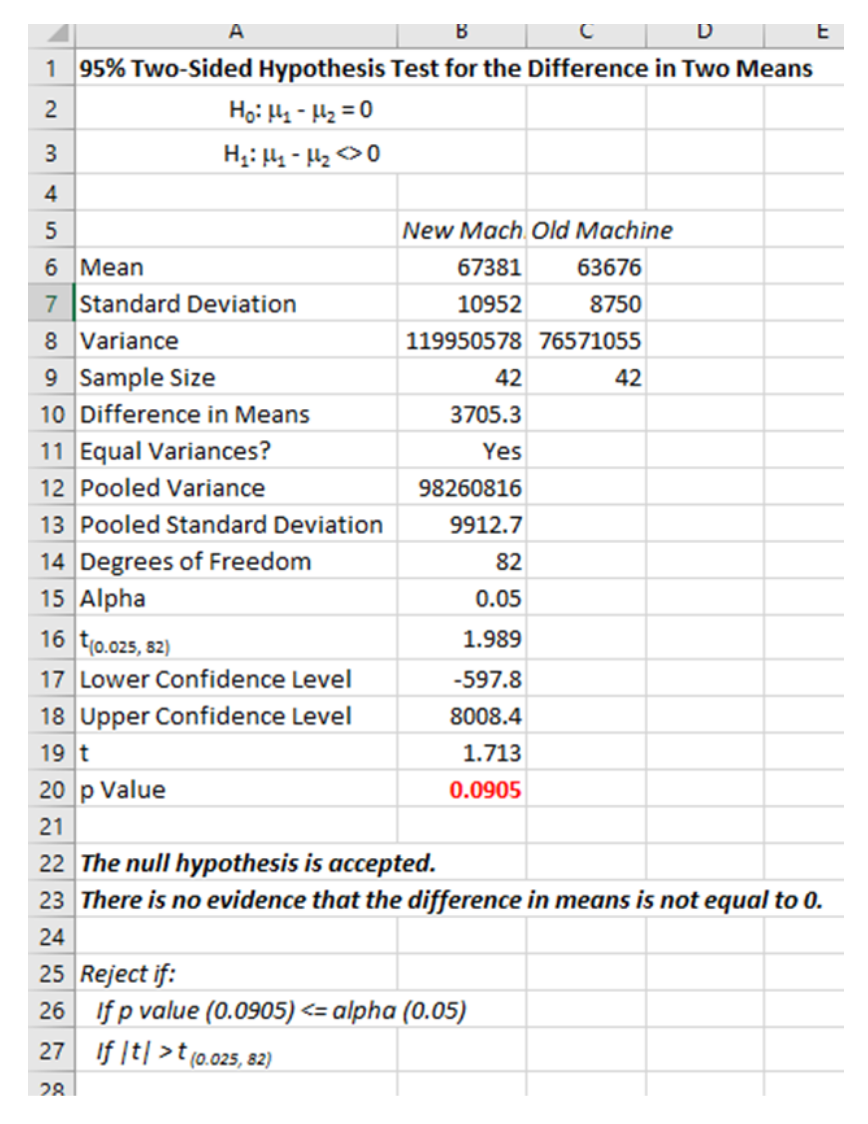While you might notice a mathematical difference between the means of two sets of sample data, is the difference statistically significant or just due to randomness? The phrase statistical significance will be used with such concepts as hypothesis testing, null and alternative hypotheses, alpha and p-value. Let’s explore this in more detail.

## Overview: What is statistical significance?

In statistics, hypothesis testing is a way for you to test the results of an experiment to see if your results are meaningful. The outcome of your hypothesis test will tell you whether your results are real or just a function of pure chance.

First, a hypothesis statement and assumption is made about the population parameter or probability distribution. This initial statement is called the null hypothesis (Ho). .It states there is no difference or change. An alternative or alternate hypothesis (Ha), is also stated, which will be the opposite of the null hypothesis or there is a statistically significant difference or change.

Typically, you will use sample data to determine whether or not you can be statistically confident in rejecting the Ho. If the Ho is rejected, the statistical conclusion is that the Ha is true. Unfortunately, there will be some inherent error in your experiment with respect to rejecting the Ho. The level at which you can accept whether an event is statistically significant is known as the significance level. The good news is you get to choose the level of acceptable error, which is called the alpha risk. This is usually selected at the .01, ,05 or .10 level of significance.

When you run your experiment and then analyze your results, your statistical software will likely provide you a p-value. This is your actual risk of wrongly rejecting the Ho. You will compare your alpha risk with your p-value and determine whether to reject the Ho or not. Your result is considered statistically significant if the p-value (actual risk) of the data analysis is less than your selected alpha (significance level and selected risk).

Keep in mind that statistical significance is different from practical significance. While your test results may be statistically significant, their business implications may not be impactful and therefore the results don’t have practical significance.

## An industry example of statistical significance

The Superintendent of a glass manufacturing company is trying to determine whether his new laminating machine is running better than his old machine. He has collected square footage data from the old machine and the new machine. Below are the results from his analysis. As you can see, the p-value of .0905 is greater than his selected level of significance (alpha) of .05. In other words, his risk of being wrong in rejecting the Ho is greater than he is willing to take so he concludes there was no statistically significant difference between the new and old machine.### How do you know if data is statistically significant?

If your p-value is less than your selected alpha you may reject the null hypothesis and claim your results are statistically significant.

### What is a common value for statistical significance

Common values for alpha for use in hypothesis testing are .01, .05 and .10.

### Is there a difference between statistical significance and significance level?

Yes. Significance levels are your selected alpha values. Statistical significance will be determined if your significance level or alpha is greater than your p-value.